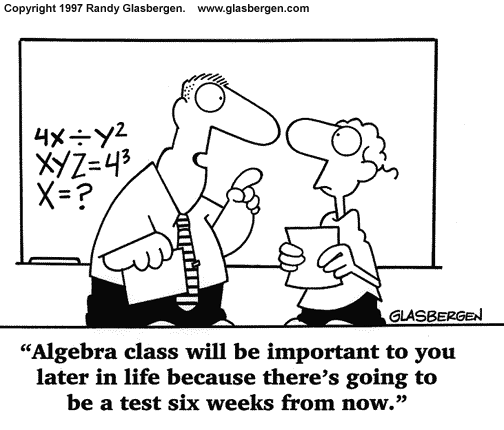## Trig RevisionRevision Sheet: Trig Revision

Complete by Thursday 28th February

Worked Solutions from class

🙂

## Trigonometry 1

Trigonometry 1: Applying trigonometric skills to solve equations

By the end of this topic you will be able to:
• convert Degrees to Radian measure and vice versa
• recall exact values in and calculate using radian measure
• translate graphs of trig functions
• solve trigonometric equations in degrees or radians

• including those involving the wave function or trigonometric formulae or identities, in a given interval

Homework: Trigonometry HW1

Due: Monday 20th December

ALL pupils should be able to Q1-4

MOST pupils should be able to complete Q5

SOME : Not applicable for HW1

DO NOT DO Q6

🙂

## Trig EquationsNew Topic: Trigonometric Equations

By the end of this topic you will be able to:

• identify related angles
• solve basic trigonometric equations
• calculate where trigonometric graphs and straight lines intersect
• work with exact vales
• work with trig identities

N5 Textbook Ch24

Homework: N5 HW – Trig Equations

😉

## Algebraic OperationsIn this topic you will learn how to:

• multiply algebraic expressions involving brackets
• factorise algebraic expressions
• factorise trinomial expressions

Class exercises: Int2 Unit 1 – Algebraic Operations

Homework: Outcome 1.4 – Algebraic Operations – Due date TBA (will be issued in August)

If you have missed any of the topic or would like extra explanation and practice please look at these revision notes from the Maths4Scotland Int2 website:

Algebra Practice

Brackets & Equations

Difference of Two Squares

Factorising Trinomials

Please comment below if you have any questions about the homework exercise or the topic.

.

## Equations & InequationsNew Topic: Equations & Inequations, MiA 4³ Ch5

By the end of this topic you will have revised your basic algebra skills including:

• gathering like terms & simplifying expressions
• substituting values (including negative numbers)
• evaluating expressions
• multiplying out brackets (single and double)
• solving equations
• You will be able to solve:

• equations involving expanding double brackets
• equations with fractions
• inequations ( etc)
• ## Brackets & EquationsNew Topic: Brackets & Equations, MiA 3³ Ch3

By the end of this topic you will have revised your basic algebra skills including:

• gathering like terms & simplifying expressions
• substituting values (including negative numbers)
• evaluating expressions
• multiplying out brackets
• You will be able to:

• multiply out (expand) double brackets
• expand squared brackets
• ## Equations and InequationsNew Topic: Equations & Inequations, MiA 4² Ch6

By the end of this topic you will be able to solve equations involving variables on both sides, equations with brackets and inequalities.